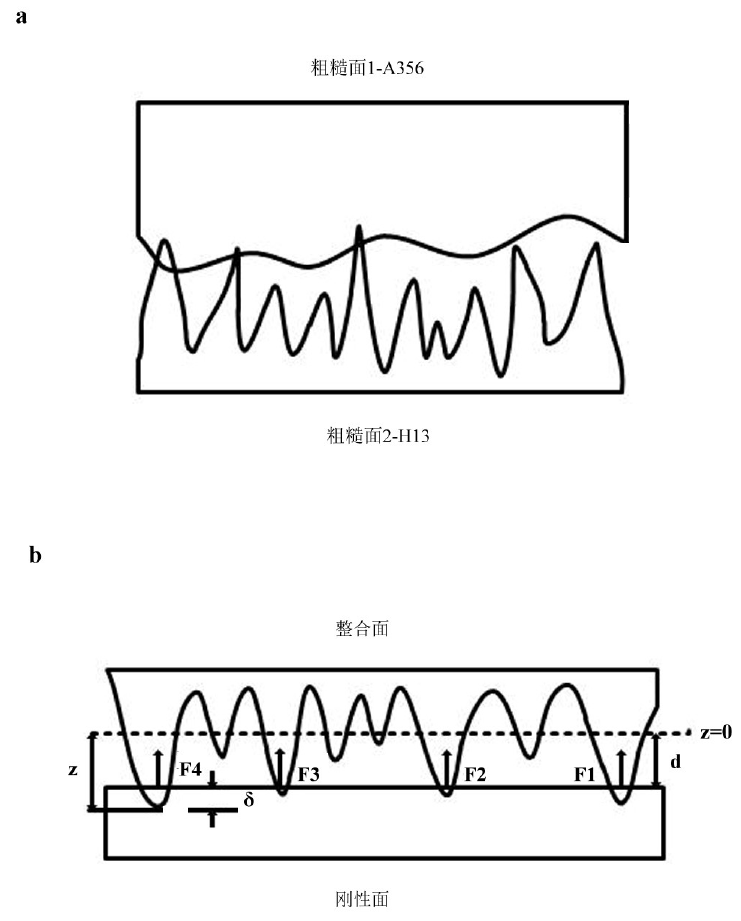# Contact analysis between two rough surfaces in Squeeze Casting

In the study, the contact between two rough surfaces is equivalent to the contact between a smooth rigid surface and a deformable rough surface, in which the deformable rough surface is defined as the conformal surface. The micro geometric parameters of the integration surface can be obtained from the micro geometric parameters of the two rough surfaces. The schematic diagram of the equivalent process is shown in Figure 1.

The geometric parameters of the integration surface can be obtained by using the micro geometric parameters of two rough surfaces according to the following relations(a) Rough surface contour curve (b) Local enlarged drawing

In the study, the micro geometric parameters of the interface are shown in Figure 2.